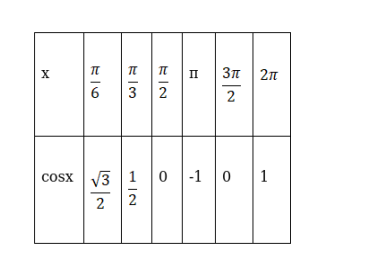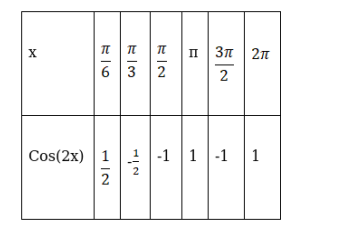# Draw the graphs of y = cos x and`
Question:

Draw the graphs of y = cos x and $y=\cos 2 x \operatorname{in}[0,2 \pi]$ on the same axes.

Solution:

For cosxFor cos(2x)The graph is:-Blue line depicts curve cos(2x)

Purple lines depict cos(x).